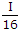# Electronics and Communication Engineering - Electromagnetic Field Theory

11.

A wave is propagated in a waveguide at frequency of 9 GHz and separation is 2 cm between walls Calculate group velocity for dominant mode.

 A. 1.8 x 108 m/sec B. 5 x 108 m/sec C. 3 x 108 m/sec D. 1.5 x 108 m/sec

Explanation:

Vg . Vp = C2

where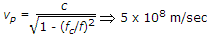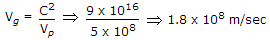.

12.

For F1 layer the maximum ionic density is 2.3 x 104 electrons per cc. The critical frequency for this layer will be

 A. 1.36 MHz B. 13.6 MHz C. 136 MHz D. 1360 MHz

Explanation:

fc = 9Nmax .

13.

Which one of the following does represents the electric field lines for the TE02 mode in the cross section of a hollow rectangular metallic waveguide?

 A.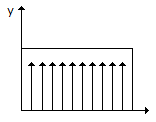B.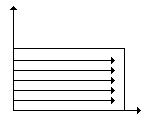C.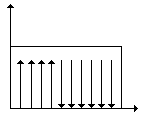D.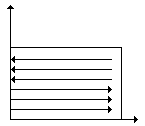Explanation:

TE02m = 0, n = 2

in x direction no propagation, only in y, and normal to the waveguide, tangential component is continuous.

14.

The velocity of electromagnetic wave in a good conductor is

 A. 3 x 108 m/s B. more than 3 x 108 m/s C. very low D. high

Explanation: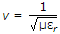, for good conductor εr >> 1.

15.

Radiation intensity of a dipole depends strongly on frequency. If at a frequency f, the intensity of radiation is 'I'. Then at a frequency of f/2, the intensity will be

 A.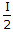B.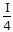C.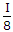D.